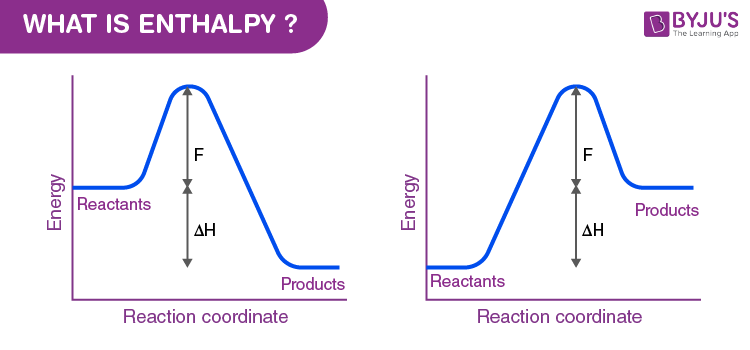# What is Enthalpy ?

## What is Enthalpy?

Enthalpy is the measurement of energy in a thermodynamic system. The quantity of enthalpy equals to the total content of heat of a system, equivalent to the system’s internal energy plus the product of volume and pressure.

Technically, enthalpy describes the internal energy that is required to generate a system and the amount of energy that is required to make room for it by establishing its pressure and volume and displacing its environment.When a process begins at constant pressure, the evolved heat (either absorbed or released) equals the change in enthalpy. Enthalpy change is the sum of internal energy denoted by U and product of volume and Pressure, denoted by PV, expressed in the following manner.

## H=U+PV

Enthalpy is also described as a state function completely based on state functions P, T and U. It is normally shown by the change in enthalpy (ΔH) of a process between the beginning and final states.

## ΔH=ΔU+ΔPV

If the pressure and temperature don’t change throughout the process and the task is limited to pressure and volume, the change in enthalpy is given by,

## ΔH=ΔU+PΔV

The flow of heat (q) at constant pressure in a process equals the change in enthalpy based on the following equation,

## ΔH=q

A relationship between q and ΔH can be defined knowing whether q is endothermic or exothermic. An endothermic reaction is the one that absorbs heat and reveals that heat is consumed in the reaction from the surroundings, hence q>0 (positive). If q is positive, then ΔH is also positive, at constant pressure and temperature for the above equation. Similarly, if the heat is released being an exothermic reaction, the heat is given to the surroundings. Hence, q<0 (negative). Therefore, ΔH will be negative if q is negative.

## Recommended Videos

### Standard Enthalpy of Formation## Frequently Asked Questions – FAQs

Q1

### Why is enthalpy useful?

Enthalpy is important because it informs us how much heat is in a system (energy). Heat is important, since from it, we can derive valuable work. An enthalpy shift shows us how much enthalpy was lost or obtained in terms of a chemical reaction, enthalpy meaning the system’s heat energy.

Q2

### What is the difference between enthalpy and internal energy?

The cumulative energy that is stored in the device is internal energy. It is the amount held by the mechanism of potential and kinetic energy. Enthalpy is specified as the amount of the system’s internal energy plus the combination of the system’s gas pressure and its length.

Q3

### What happens when enthalpy is positive?

A heat absorption reaction is endothermic. Its enthalpy will be positive, and its surroundings will cool down. This reaction (negative enthalpy, heat release) is exothermic. When the reaction happens, due to the gain in heat the device emits, the atmosphere may rise in temperature.

Q4

### What is enthalpy of chemical reaction?

The bonds between atoms can dissolve, reform or both during chemical reactions to either absorb or release energy. The heat absorbed or emitted under constant pressure from a device is referred to as enthalpy, and the reaction enthalpy is the change in enthalpy arising from a chemical reaction.

Q5

### Is enthalpy always greater than internal energy?

The heat energy produced is often used in thermodynamics to improve the system’s energy or to do some useful work. The energy associated with an open system is called enthalpy, which is often greater than or equal to internal energy.

To know more about a difference between enthalpy and entropy along with examples enthalpy equation and real-time examples at BYJU’S.

Test Your Knowledge On What Is Enthalpy!